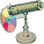Flash Bestiary / Natural Phenomena / Lightning Generator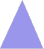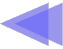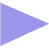# Lightning Generator

This movie simulates lightning bolts using midpoint displacement.

Midpoint Displacement is an algorithm commonly used for generating fractal terrain. You'll notice that the shape of the lightning bolts resembles the side of an idealized mountain range.

The recursive routine lightning(), excerpted below, does all the work.

function drawLightning(x1,y1,x2,y2,displace)
{
if (displace < curDetail) {
graf.moveTo(x1,y1);
graf.lineTo(x2,y2);
}
else {
var mid_x = (x2+x1)/2;
var mid_y = (y2+y1)/2;
mid_x += (Math.random()-.5)*displace;
mid_y += (Math.random()-.5)*displace;
drawLightning(x1,y1,mid_x,mid_y,displace/2);
drawLightning(x2,y2,mid_x,mid_y,displace/2);
}
}

You pass it the coordinates of a line segment (x1,y1,x2,y2) and a displacement value. It computes the midpoint of the line (mid_x,mid_y), and then displaces it by a random value. The random value is scaled by the displacement value, which is reduced by half each time we subdivide the line.The first (big) subdivision gets a big displacement, and the smaller subdivisions get smaller displacements (the displacement value gets divided by 2 for each level of recursion). This is what makes it 'fractally'.

When the displacement value falls below some arbitrary minimum (specified by the 'detail' slider) we draw the line segment. This is the line that says

if (displace < curDetail)

If you change the curDetail to a large value, you'll get fewer (and longer) line segments and the lightning will render faster, but will look less 'realistic'.

UPDATE: The source code below now contains an Actionscript 3 version, in addition to the original Flash MX code.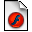Download the flash project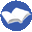Jim's Favorite Actionscript Books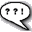Ask Jim about ActionscriptNext: Windy Grass

Having trouble opening the project? You may need to Upgrade to Flash CS3